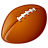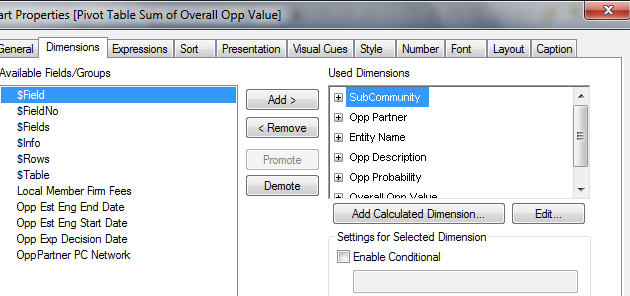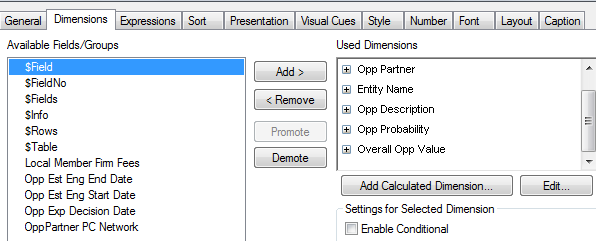# QlikView App Dev

Discussion Board for collaboration related to QlikView App Development.

Announcements
Read about the latest Qlik Community enhancements on the Community News blog!
cancel
Showing results for
Did you mean:Not applicable

## division with two if statement

Hi everyone,

The calculation in my pivot table is actually off.

I am trying to divide this expression, numerator: =IF(TODAY()>[Opp Exp Decision Date], ([Local Member Firm Fees]*[Opp Probability]/100)/295*0.5,[Local Member Firm Fees]*[Opp Probability]/100/295)

with denominator: =IF(((([Opp Est Eng End Date]-[Opp Est Eng Start Date])/30)=0),1,([Opp Est Eng End Date]-[Opp Est Eng Start Date])/30)

the two expressions alone generate the correct values, but not when I link them in division with this expression: =IF(TODAY()>[Opp Exp Decision Date], ([Local Member Firm Fees]*[Opp Probability]/100)/295*0.5,[Local Member Firm Fees]*[Opp Probability]/100/295)/IF(((([Opp Est Eng End Date]-[Opp Est Eng Start Date])/30)=0),1,([Opp Est Eng End Date]-[Opp Est Eng Start Date])/30)

the number is a little off. Can somebody please guide me on how to link the two with division?

11 RepliesCreator II

Hi Can you share a sample app to highlight your issue?MVP

Did you try using my Sum(Aggr(...)) logic? I feel that should workNot applicable
Author

can you type out the whole expression for me? I tried using the sum aggr expression and I see the expression is in error.

=sum(aggr(IF(TODAY()>[Opp Exp Decision Date], ([Local Member Firm Fees]*[Opp Probability]/100)/295*0.5,[Local Member Firm Fees]*[Opp Probability]/100/295))),IF(((([Opp Est Eng End Date]-[Opp Est Eng Start Date])/30)=0),1,([Opp Est Eng End Date]-[Opp Est Eng Start Date])/30)))MVP

Can you provide me the list of dimensions used in your pivot table?Not applicable
Author

I would like to provide a sample excel file, where do I attach it?MVP

I just want to know the names of the dimensions.Not applicable
Author

dimension used for the numerator:these are the used dimensions. Let me know if this is what you need.Not applicable
Author